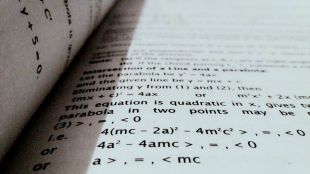Search IntMath
Close

# 1. Addition and Subtraction of Algebraic ExpressionsBefore we see how to add and subtract integers, we define terms and factors.

## Terms and Factors

A term in an algebraic expression is an expression involving letters and/or numbers (called factors), multiplied together.

### Example 1

The algebraic expression

5x

is an example of one single term. It has factors 5 and x.

The 5 is called the coefficient of the term and the x is a variable.

### Example 2

5x + 3y has two terms.

First term: 5x, has factors 5 and x

Second term: 3y, has factors 3 and y

The 5 and 3 are called the coefficients of the terms.

### Example 3

The expression

3x^2 - 7ab + 2esqrt(pi)

has three terms.

First term: 3x^2 has factors 3 and x2

Second term: -7ab has factors -7, a and b

Third Term: 2esqrt(pi); has factors 2, e, and sqrt(pi).

The 3, -7 and 2 are called coefficients of the terms.

## Like Terms

"Like terms" are terms that contain the same variables raised to the same power.

### Example 4

3x2 and 7x2 are like terms.

### Example 5

-8x2 and 5y2 are not like terms, because the variable is not the same.

Important: We can only add or subtract like terms.

Why? Think of it like this. On a table we have 4 pencils and 2 books. We cannot add the 4 pencils to the 2 books - they are not the same kind of object.

We go get another 3 pencils and 6 books. Altogether we now have 7 pencils and 8 books. We can't combine these quantities, since they are different types of objects.

Next, our sister comes in and grabs 5 pencils. We are left with 2 pencils and we still have the 8 books.

Similarly with algebra, we can only add (or subtract) similar "objects", or those with the same letter raised to the same power.

### Example 6

Simplify 13x + 7y − 2x + 6a

13x + 7y − 2x + 6a

The only like terms in this expression are 13x and -2x. We cannot do anything with the 7y or 6a.

So we group together the terms we can subtract, and just leave the rest:

(13x − 2x) + 6a + 7y

= 6a + 11x + 7y

We usually present our variables in alphabetical order, but it is not essential.

### Example 7

Simplify −5[−2(m − 3n) + 4n]

Go back to Order of Operations if you are not sure what to do first with this question.

−5[−2(m − 3n) + 4n]

The square brackets [ ] work just the same as round brackets ( ). We could have used curly brackets { } here as well.

The first thing we do is expand out the round brackets inside.

−2(m − 3n) = −2m + 6n

The negative times negative in the middle gives positive 6n.

Now add the 4n in the square brackets:

[−2m + 6n + 4n] = [−2m + 10n]

Remembering the −5 out front, our problem has become:

−5[−2m + 10n] = 10m − 50n

Taking each term one at a time, what we did was:

−5 × −2m = 10m (Two negative numbers multiplied together give a positive); and

−5 × 10n = −50n (Negative times positive gives negative)

Go back to the section on Integers if you are not sure about multiplying with negative numbers.

−5[−2(m − 3n) + 4n] = 10m − 50n

Note:

The fancy name for round brackets ( ) is "parentheses".

The fancy name for square brackets [ ] is "box brackets".

The fancy name for curly brackets { } is "braces".

### Example 8

Simplify −[7(a − 2b) − 4b]

−[7(a − 2b) − 4b]

= −[7a − 14b − 4b]

= −[7a − 18b]

= −7a + 18b

## Problem SolverThis tool combines the power of mathematical computation engine that excels at solving mathematical formulas with the power of GPT large language models to parse and generate natural language. This creates math problem solver thats more accurate than ChatGPT, more flexible than a calculator, and faster answers than a human tutor. Learn More.